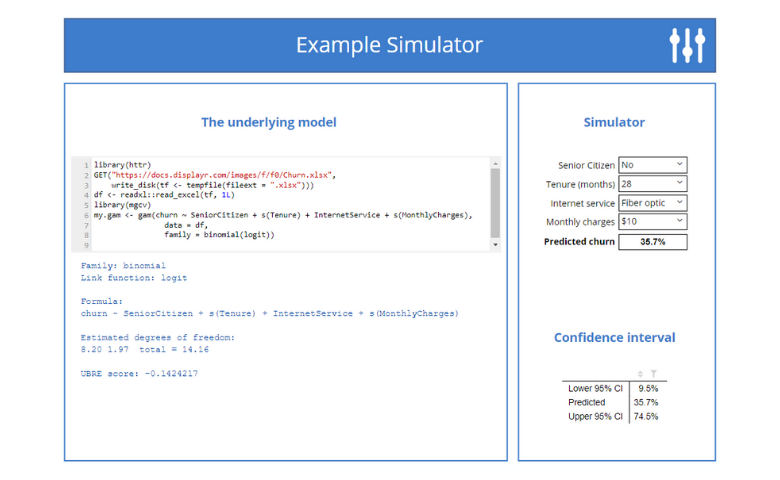# Blog.

## Using R.How to Create a Correlation Matrix in R
23 October 2018 | by Chris Facer

A correlation matrix is a table of correlation coefficients for a set of variables used to determine if a relationship exists between the variables. TheBuilding Online Interactive Simulators for Predictive Models in R
26 September 2018 | by Tim Bock

Learn how to create interactive simulators, where users can manipulate predictor variables and see how the predictions change.Linear Discriminant Analysis in R: An Introduction
11 October 2017 | by Jake Hoare

How does Linear Discriminant Analysis (LDA) work and how do you use it in R? This post answers these questions and provides an introduction to LDA.Automatically Fitting the Support Vector Machine Cost Parameter
18 July 2017 | by Jake Hoare

I show how to automatically fit the Support Vector Machine cost parameter by automating the manual search for the optimal cost.